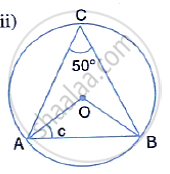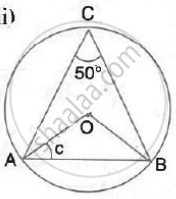Share

In the Following Figures, O is the Centre of the Circle. Find the Values of A, B, C and D. - ICSE Class 10 - Mathematics

ConceptChord Properties - Chords Equidistant from the Center Are Equal (Without Proof)

Question

In the following figures, O is the centre of the circle. Find the values of a, b, c and d.

Solution∠AOB = 2 ∠AOB = 2×50°= 100°
(Angle at the centre is double the angle at the circumference subtended by the same chord)
Also, OA = OB
⇒  ∠OBA = ∠OAB = C
∴  c = (180° - 100°)/2 = 40°

Is there an error in this question or solution?

Video TutorialsVIEW ALL 

Solution In the Following Figures, O is the Centre of the Circle. Find the Values of A, B, C and D. Concept: Chord Properties - Chords Equidistant from the Center Are Equal (Without Proof).
S